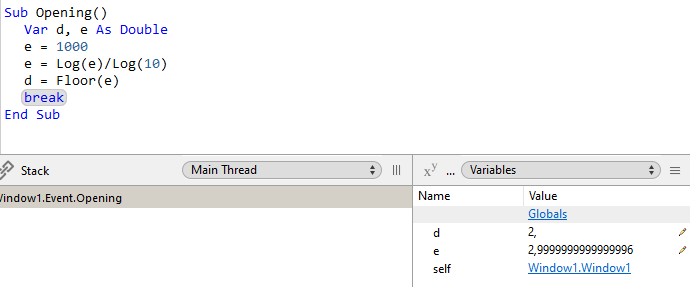# Error with FLOOR?

Hello my friends,
I have been using for a long time a basic function which allows to calculate the number of digits in an integer.
Yesterday I came across a case that seemed incorrect to me so I did a test and indeed it seems that there is an error calling the FLOOR function.

``````  Dim Result As Integer
Dim Division As Double
Dim a As Integer

a = 1000

Division = Log(a)/Log(10)

Result = Floor( Log(a)/Log(10) ) + 1

t_Out.WriteLine "a : " + Str(a)
t_Out.WriteLine "Log(a) : " + Format( Log(a), "#.#########" )
t_Out.WriteLine "Log(10) : " + Format( Log(10), "#.#########" )
t_Out.WriteLine "Division : " + Format( Division, "#.#########" )
t_Out.WriteLine "Floor : " + Format( Floor(Division), "#.#########" )
t_Out.WriteLine "nb: " + Str( Result )
t_Out.WriteLine "Test 3.005: " + Str( Floor(3.005) )
t_Out.WriteLine "Test 3.: " + Str( Floor(3.) )
``````

The results :
a : 1000
Log(a) : 6,907755279
Log(10) : 2,302585093
Division : 3,
Floor : 2, Here is the error.
nb: 3
Test 3.005: 3
Test 3.: 3

Am I making a mistake somewhere or is there really a rounding error?
How can we get around this problem?
Thank you.

Imprecision edge case?2 Likes

If I understand correctly it’s rather annoying because it doesn’t happen for example with Excel or another programming language that I use.
I had to convert the number to text and calculate the length of the string, which is less elegant.

What is the value of `division` without using the Format command?

My guess is that it’s really close 3 but requires more than 9 decimals to express.

Remember that Format and Str can cause their own rounding if you don’t provide enough decimal places in the formatting string.

Also, try this:

``````Dim a as Double
a = 1000.0
``````

Relying on the framework to do that for you may be where your issue lies.

My guess is that this is related to using floating point arithmetic. I imagine Excel doesn’t use it and maybe the other programming language doesn’t.

If the accuracy of the calculations is important then you probably need to use some kind of fixed precision library / plugin such as the ones available from Bob Delaney and MBS.

With format you are rounding, change your code to:

``````system.debuglog "Division : " + Format( Division, "#.########################" )
``````

and you will get expected results (for floating point arithmetic, see above):

Division : 2.99999999999999955591079
Floor : 2.

A workaround to fix such edge rounding case would be:

Log(a)/Log(10)+1e-15

So 2.9999999999… gets 3.00000000…